Courses

# Hermite and Laguerre Special Functions (Part - 3)- Mathematical Methods of Physics, UGC -NET Physics Physics Notes | EduRev

## Physics for IIT JAM, UGC - NET, CSIR NET

Created by: Akhilesh Thakur

## Physics : Hermite and Laguerre Special Functions (Part - 3)- Mathematical Methods of Physics, UGC -NET Physics Physics Notes | EduRev

The document Hermite and Laguerre Special Functions (Part - 3)- Mathematical Methods of Physics, UGC -NET Physics Physics Notes | EduRev is a part of the Physics Course Physics for IIT JAM, UGC - NET, CSIR NET.
All you need of Physics at this link: Physics

Exercises

13.2.1 Show with the aid of the Leibniz formula that the series expansion of Ln (x ) (Eq. (13.60)) follows from the Rodrigues representation (Eq. (13.59)).

13.2.2 (a) Using the explicit series form (Eq. (13.60)) show that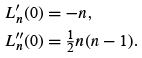(b) Repeat without using the explicit series form of Ln (x ).

13.2.3 From the generating function derive the Rodrigues representation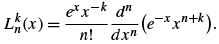13.2.4 Derive the normalization relation (Eq. (13.79)) for the associated Laguerre polynomials.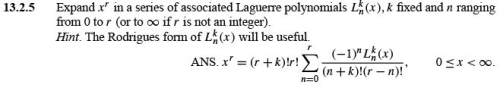13.2.6 Expand e−ax in a series of associated Laguerre polynomials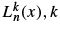ﬁxed and n ranging from 0 to ∞.

(a) Evaluate directly the coefﬁcients in your assumed expansion.

(b) Develop the desired expansion from the generating function.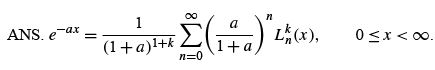13.2.7 Show that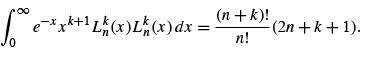Hint. Note that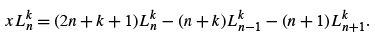13.2.8 Assume that a particular problem in quantum mechanics has led to the ODE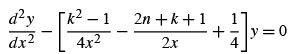for nonnegative integers n, k . Write y(x) as

y(x) = A(x)B(x)C(x),

with the requirement that

(a) A(x ) be a negative exponential giving the required asymptotic behavior of y(x) and

(b) B(x ) be a positive power of x giving the behavior of y(x) for 0 ≤ x ≪ 1.

Determine A(x ) and B(x ). Find the relation between C(x) and the associated Laguerre polynomial.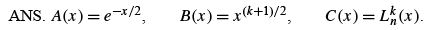13.2.9 From Eq. (13.91) the normalized radial part of the hydrogenic wave function is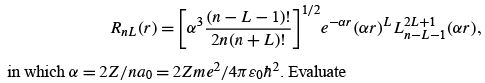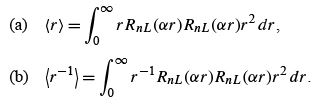The quantity { r } is the average displacement of the electron from the nucleus, whereas {r −1} is the average of the reciprocal displacement.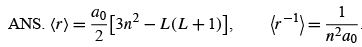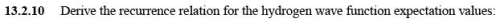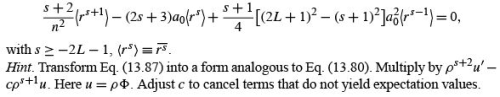13.2.11 The hydrogen wave functions, Eq. (13.91), are mutually orthogonal, as they should be, since they are eigenfunctions of the self-adjoint Schrödinger equation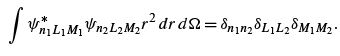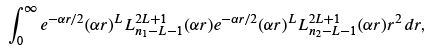which appears to match Eq. (13.83) and not the associated Laguerre orthogonality relation, Eq. (13.79). How do you resolve this paradox?

ANS. The parameter α is dependent on n. The ﬁrst three α,previously shown, are 2Z/ n1 a0 . The last three are 2Z/ n2 a.For n1 = n2 Eq. (13.83) applies. For n1 = n2 neither Eq. (13.79) nor Eq. (13.83) is applicable

13.2.12 A quantum mechanical analysis of the Stark effect (parabolic coordinate) leads to the ODE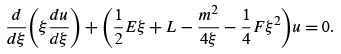Here F is a measure of the perturbation energy introduced by an external electric ﬁeld.
Find the unperturbed wave functions (F = 0) in terms of associated Laguerre polynomials.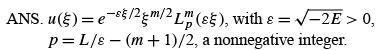13.2.13 The wave equation for the three-dimensional harmonic oscillator is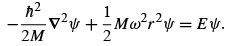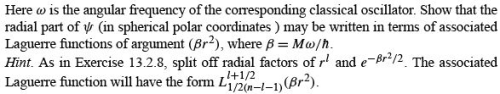13.2.14 Write a computer program that will generate the coefﬁcients as in the polynomial form of the Laguerre polynomial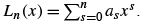13.2.15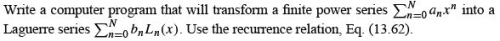13.2.16 Tabulate L10 (x ) for x = 0.0(0.1)30.0. This will include the 10 roots of L10 . Beyond x = 30.0,L10 (x ) is monotonic increasing. If graphic software is available, plot your results.

Check value. Eighth root = 16.279.

13.2.17 Determine the 10 roots of L10 (x ) using root-ﬁnding software. You may use your knowledge of the approximate location of the roots or develop a search routine to look for the roots. The 10 roots of L10 (x ) are the evaluation points for the 10-point Gauss–Laguerre quadrature. Check your values by comparing with AMS-55, Table 25.9.

13.2.18 Calculate the coefﬁcients of a Laguerre series expansion (Ln (x ), k = 0) of the exponential e−x . Evaluate the coefﬁcients by the Gauss–Laguerre quadrature (compare Eq. (10.64)). Check your results against the values given in Exercise 13.2.6.
Note. Direct application of the Gauss–Laguerre quadrature with f(x )Ln (x )e−x gives poor accuracy because of the extra e−x . Try a change of variable, y = 2x , so that the function appearing in the integrand will be simply Ln (y /2).

13.2.19 (a) Write a subroutine to calculate the Laguerre matrix elements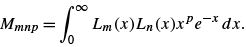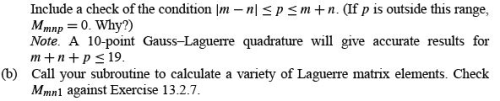13.2.20 Write a subroutine to calculate the numerical value of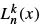for speciﬁed values of n, k , and x . Require that n and k be nonnegative integers and x ≥ 0.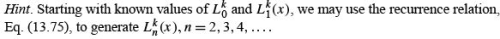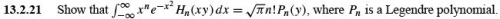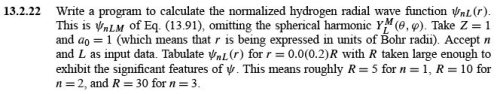159 docs

,

,

,

,

,

,

,

,

,

,

,

,

,

,

,

,

,

,

,

,

,

,

,

,

;